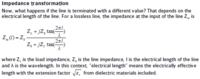# signal integrity Quesition in PCB

Status
Not open for further replies.

#### eng_ahmed_osama

##### Junior Member level 3
My question is about in high speed design the wire becomes transmission lines if the wave length is bigger than the path of the wire connection , but in the pcb the wire connection become much bigger than the wave length what happens now still wire or treats as TL

Thanks

if the wave length is bigger than the path of the wire connection

It must be treated as a transmission line if the wave length is not much bigger than the path length. Or in other words ... if the path length is longer than 1/20 wavelength or so.

For PCB, it is the same: when the line is longer, we start to see a difference from an ideal connection. The wavelength in this calculation is the effective wavelength calculated with the effective dielectric constant, so it is shorter than the wavelength in air.

But what about the length of the wire Lamda/2 (half wave length) actually i don't understand the effect of wire length and wave length . is their any easy tutorial for this relation .

What actually you want to understand.....Whenever the Wire-length/trace length is comparable(this can be length/6 or some references give l/10..etc) to Wavelength(calculated based on Rise time of the driver) of the wave travelling we need to consider that wire/trace as transmission line and properly simulate for the reflections... etc. same thing people explain in-terms of round trip delay and rise time. Why we are considering wavelength is when trace length is very small considered to wavelength/10 or 20 then over the length of the wire E or H(Electro Mangnetic fields) will not have much changes.So, there is no need to take special care of the trace....

•matrixofdynamism

### matrixofdynamism

Points: 2
It is a continuos effect, not a sudden change in behaviour.
For smaller trace length, the transmission line effects are smaller. You can use transmission line calculations at low frequency, and results are valid, but the difference to "DC mathematics" is too small to see an effect. That is why we only use transmission line calculation where the effect is large enough to notice.

- - - Updated - - -

Here is the math to calculate the input impedance Zin for a transmission line that is terminated with ZL, using a line impedance Z0. You can see that for very small l/lambda, we always get Zin=ZL independent of the line. That is the "short line" case where transmission line effects are too small to see an effect.•matrixofdynamism

### matrixofdynamism

Points: 2

A fun way to check this out is by using "Bandwidth Calculator & Max PCB Trace Length Calculator" from Saturn PCB design tool

https://www.saturnpcb.com/pcb_toolkit.htm

This gives you an image of how the signal behaves depending on its frequency and trace length.

•matrixofdynamism

### matrixofdynamism

Points: 2
Firstly to determine WHAT is a high speed signal you need to know its RISE TIME, this will give you the knee frequency, you will then know what frequency content you have cater for...
Or treat all digital as critical and always use transmission line calculation if required.

•matrixofdynamism

### matrixofdynamism

Points: 2
Firstly to determine WHAT is a high speed signal you need to know its RISE TIME, this will give you the knee frequency, you will then know what frequency content you have cater for...

Rise time is digital designer's language. The OP had asked about wavelength, so this looks like an analog signal of well know frequency (more or less narrowband, no harmonics to consider).

•matrixofdynamism

Points: 2